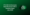# VTU Exam Papers BE-CS 3rd Semester Data Structures with C July 08VTU CSE 3rd Semester Data Structures with C Question Papers July 08: Every semester has an important role to shape Computer Science & Engineering Career.

To score the better mark in the Data Structures with C semester exam, you must solve previous exam papers. It will give you information about the important chapters and concepts to be covered in all chapters.

Here we are providing you the complete guide on VTU CSE 3rd Semester Data Structures with C Question Papers July 08.

## VTU CSE 3rd Semester Data Structures with C Question Papers July 08

You must have Data Structures with C Question Papers along with the latest Computer Science 3rd sem Syllabus to enhance your semester exam preparation.

Here you can check the VTU CSE 3rd Semester Data Structures with C Question Papers July 08

#### SET-A

Note : Answer any FIVE full questions.

1 a. If m and n have been declared as integers and pi and p2 as pointers to integers,

then state errors, if any in the following statement:

i) m = p1/p2

ii) P2= n

iii)*p1= &n

iv) p1 = &p2

v) m=p1-p2

b. Given the following declarations intx = 10, y = 10, res; int *pi = &x, *p2 = &y;

What is the value of res for following expressions: i) res = (*pi)++;

(ii) res —-(*p2);

(iii) res = *pi + (*P2)-S . iv) res =++(*p2) – *pi;

(v) res = -H-x + y++;

c. Define: i) Nested structures ii) Array of structure. Write a program by making use of the above concept to store student information and display the same. (10 Marks)

2 (a)What do you mean by dynamic memory allocation? Explain any three functions that support dynamic allocation.

b.What is preprocessor directive? Explain any two operations using preprocessor directive.

c. Explain the following function with suitable example:

i) fseek( )

ii) ftell ( )

iii) rewind( )

Write a program to count the number of characters, blanks, lines and tabs in a given input file.

3. a. Design a method for keeping two stacks (grow towards each other) within a single linear array so that neither stack overflows until all of memory is used and an entire stack is never shifted to a different location within the array. Write C routines pushl, push2, popl, pop2, display 1, display2 to manipulate the two stacks.

b. Write a program to accept input as a characters string of operators and operands representing a postfix expression and, to create the fully parenthesized infix form of the original postfix. For example AB+ would be transformed into (A+B) and AB+C- would be transformed into ((A+B)-C).

4.a. Determine what the following recursive C function computes. Write an iterative function to accomplish the same purpose.int func (int n) { if(n= -0) return 0; retum (n+func(n-1));} And also distinguish between the recursive function and iterative function.

b. What is priority queue? Mention the different types of priority queues. Explain briefly the problems arise while inserting and deleting an item from the priority queue. Write a C program to implement priority queue.

5 a. What are the advantages and disadvantages of representing a group of items as an array versus a linear linked list?

b. Implement the routines insert, delete and display using a dynamic storage implementation of a linked queue.

c. Write a C function to concatenate two lists.

6 a. Define the following and give one example for each:

i) Binary tree

ii) Strictly binary tree

(iii) Complete binary tree

b. Write recursive functions to determine:

(vi) The number of nodes in a binary tree

(vii) The height of a binary tree.

c. Write a C routine to evaluate postfix expression using an expression tree.

7 a. Explain how an index sequential search is used to search for an element. Write down the advantages and disadvantages of index sequential search.

b Explain address calculation sort: 25, SI, 48, 37, 12, 92, 86, 33 Show the steps to sort the above elements.

C .Write short notes:

a. Hashing